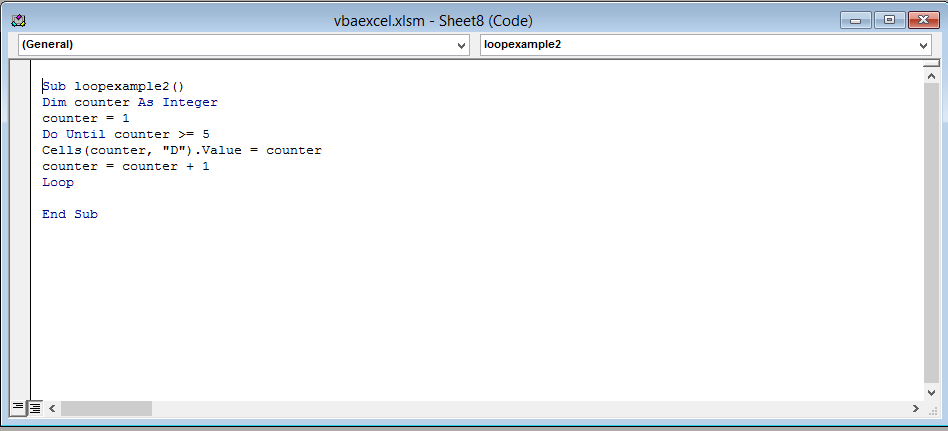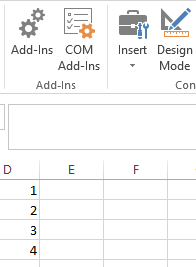#### Do Until Loop in Excel VBA

In this Excel VBA tutorial lesson, we are going to learn about programming DO UNTIL loops. First, we are going to learn what loops are and why they are necessary. Then we are going to learn how to use a DO UNTIL LOOP.

## Types of VBA loops

Note: In Excel VBA, there are two types of DO LOOPS: DO WHILE and DO UNTIL. Our focus will be on the DO UNTIL loop in this article.

Consider the following code:

```Dim numbcount As Integer
numbcount = 1 + 2 + 3 + 4 + 5 + 6 + 7 + 8 + 9 + 10```

This code is used to add the numbers from 1 to 10. The result is then stored in the variable numbcount. Now this looks pretty easy and simple right now. But now suppose instead of the first ten number you want to add numbers 1 to 1000, now are you going to write all the numbers and then add them? Of course you can, but that would become tedious. So what do we do? We use the handy programming tool called “Loop”.

Loop as the names sound is something that goes on and on until you tell it to stop (this is called a “condition”). You set a starting number for your loop, an end condition, and a way to get from the starting number to the end condition. In VBA, there are four types of loops to choose from: For loops, for each loop, Do Loops, and While loops. For now, we will focus on the DO UNTIL loop.

First let’s look at the syntax of the DO UNTIL LOOP:

```Do Until [condition]
Loop```

Explanation: First you declare the DO UNTIL loop by writing “Do Until” followed by square brackets in which you write a condition. This condition will be used to end your DO UNTIL loop.

Here is an example for you to understand the syntax better:

```Do Until counter > 5
counter = counter + 1
Loop```

Explanation: This code will increase the value of a variable called count by 1 and then store the value in the same variable count. This loop will keep on going again and again until the condition statement is true, meaning until the value of count is less than equal to 5.

Now let’s see how to use the Do UNTIL loop.

## The ribbon

Right-click anywhere on the ribbon and select “Customize the Ribbon” from the drop-down list.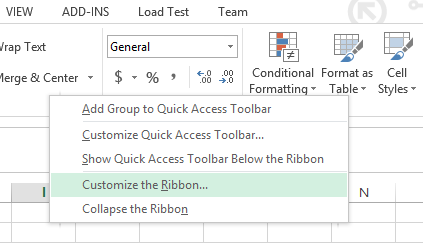An option dialog box will open. Click on the DEVELOPER check box (it is under “Customize the Ribbon Main Tabs”) and press ok.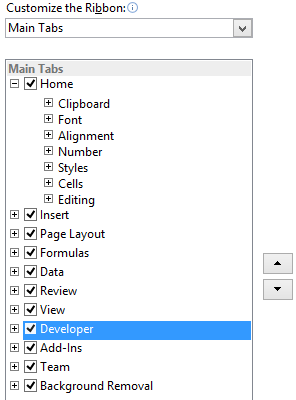The developer tap is now visible and is present next to the view tab on the top menu bar. Click on the Developer tab and select “View Code”.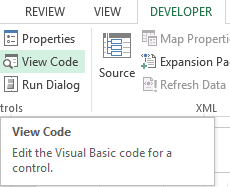## The code

A new window (Visual Basic Editor) will open which will have a dialog box in the center. You will write the code in the dialog box.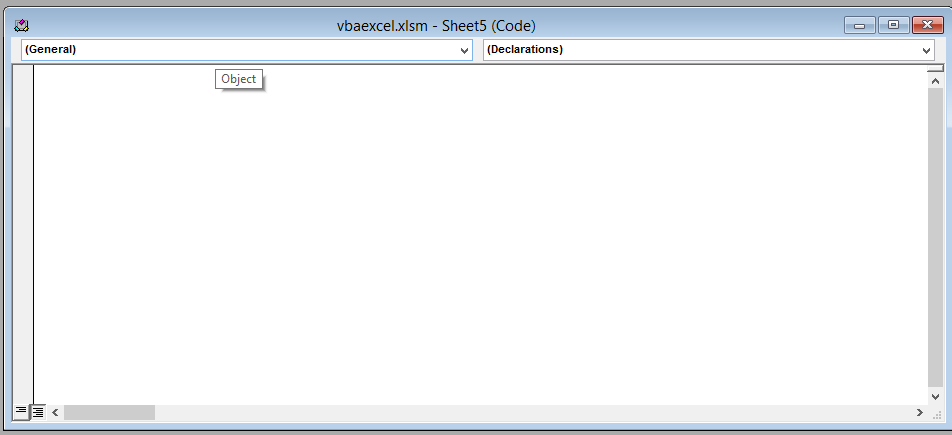Write the following line of code in the dialog box.

```Sub loopexample2()
Dim counter As Integer
counter = 1
Do Until counter >= 5
Cells(counter, "D").Value = counter```

After writing the code, close the window by clicking on the cross(x) icon on the upper right side of the screen. Don’t worry, Excel won’t close.